• 검색 결과가 없습니다.

# IMPLEMENTATION OF WEIGHTED DAMPED LEAST SQUARES INVERSE KINEMATICS

N/A
N/A
Protected

Share "IMPLEMENTATION OF WEIGHTED DAMPED LEAST SQUARES INVERSE KINEMATICS "

Copied!
68
0
0
더 보기 ( 페이지)

전체 글

## INTRODUCTION

### Background

B} The robot base coordinate frame is located at the bottom of the robot manipulator, also known as link zero. E_0} End effector_0 coordinate frame whose origin is located at the endpoint of the last link and is defined relative to the robot base coordinate.

### Motivation

The goal of workcell calibration is to define the true transformations (in the real workcell) between the robot workcell coordinate frames. The next step is to regenerate the robot trajectory by considering the dimensional variations obtained in the first step.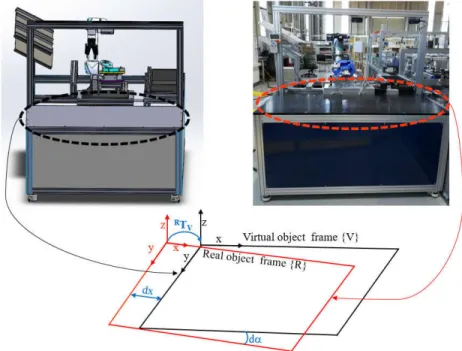Figure 1.4 Dimensional variations between the virtual and real work object frames

### Objective

Since the transformation matrix describing the frame of the virtual work object with respect to the base frame of the robot { _ } is briefly extracted from the CAD model), it can be calculated by composite transformations. The robot's manipulability index is used to determine how close the robot is to the singularity and the resulting deviation of the robot from the singularity.

### Overview

The damping factor allows the continuity of the inverse kinematics solution, which can be tuned to the manipulatability index of the robot manipulator. In (a) the robot path deviates from the desired path due to the damping factor and in (b) the robot manipulator moves away from the singular region due to the damping factor.

## LITERATURE SURVEY

### Robot Programming

Automatic programming systems require little or no direct user involvement in the development of the robot program. Online programming does not require calibration because dimensional errors do not affect the repeatability of the robot (Duelen and Schröer 1991).

### Kinematic modelling and forward kinematics

Because the robot is not present during programming, these methods are often called offline programming systems. With these systems, programming takes place while the robot system is running, which is why they are also called online programming systems. Offline programming is usually done on a CAD model of the robot and its peripherals; and sometimes there are dimensional variations between the physical system and the CAD model.

The robot programs generated in this way must be calibrated as these dimensional errors will affect the robot's absolute position accuracy. The movement of the robot is derived from the composition of each link's movement with respect to the previous one. A minimum of six joints is required for the robot to achieve any position and orientation in its workspace.

In other words, the number of degrees of freedom of the manipulator robot is more than the number of degrees of freedom of its end effector.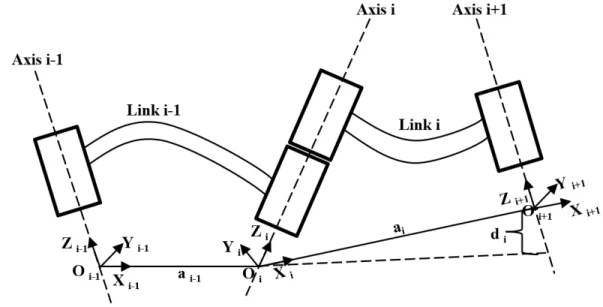Figure 2.2 Modified Denavit-Hartenberg convention The coordinate frame attached to each link can be defined as:

### Inverse kinematics

• Resolved Motion Rate Control
• Jacobian Transpose
• Jacobian Pseudoinverse
• Damped Least Square Inverse

Analytical methods provide solutions of joint variables with respect to the Cartesian coordinates of the end-effector. The solution to equation (2.9) is not unique and usually depends on the initial state of the joint coordinates. With RMRC, this is achieved by calculating the Cartesian coordinates of the end effector at each time ( ) and from that obtaining the joint coordinates using equation (2.8).

Their method also provided a measure of the degree of recovery of the manipulability index around features. Jacobian transposition provides an intuitive inverse kinematics solution based on Lyapunov's theorem for nonlinear systems (Khalaji and Moosavian 2015). At singularities, the value of the manipulability index decreases to zero and the end effector loses one or more degrees of freedom.

Another method proposed by (Chiaverini, Egeland et al. 1991) adjusts the manipulability index in terms of the minimum singular value.

### Robot Workcell Calibration

Tao, Mustafa et al. 2015) proposed a method that calculates the actual relative positions of the robot TCP and the work object frame in the sensor coordinate frame. With accurate measurements, the robot TCP is directed to follow the desired robot path in the work object coordinate frame. Liu, Shen et al. 2008) designed a line-based calibration scheme that calibrates the transformation matrix connecting the robot base frame to the work object frame.

Then, a least square method and a quaternion algorithm are used to determine the transformation matrix that relates the work object frame to the robot base frame. Once the robot manipulator is kinematically calibrated, it can be used as a measuring tool for the calibration of the work object. Two sets of matching position data points are needed to obtain the transformation matrix of the work object relative to the robot base.

The limit of the manipulability index W = 0.001 and = 0.1 was used to check the feasibility of the path in the real work object frame.

## PATH REGENERATION

### Transformation Matrix Computation

The first set of points is measured relative to the work object, while the second set of points is measured with the robot and the values ​​are read from the teaching pendant. The work object frame is defined by measuring three points as shown in Figure 3.1, by choosing point {1} as the origin of the work object frame, the other two points are defined relative to this origin. Thus, two datasets are obtained that will be used to calculate the transformation matrix between the real work object frame and the robot base frame { _ }. describes the orientation and position between the two frames.

A linear regression method applied to the corresponding data points is used to determine the transformation matrix. By putting the results in matrix form, the values ​​of the transformation matrix can be expressed as The transformation matrix between the robot base frame and the virtual work object frame { _ } is obtained from the CAD model, to calculate the transformation matrix of the real work object relative to virtual work object frames in short ) by composite transformations.

Given a path in the virtual work object frame VPM, a corresponding path in the real work object RPM can be obtained by equation (3.8).

### Path Feasibility Check and Regeneration

• Manipulability Index
• Closed Loop Inverse Kinematics

This is known as a robotic manipulator kinematic singularity, where the robotic manipulator cannot produce motion in one or more degrees of freedom of the end effector. In this way, a feasible and continuous solution is ensured at the cost of a robot end effector deviating from the desired path. Near the singularity, the robotic manipulator exhibits jerky motion because a small change in the motion of the end effector will cause large changes in the joint angles.

The damping factor serves as a means to reduce these lock angle variations causing minimal errors in the end effector motion. The manipulability index is a value that determines the ability of a robot manipulator to change the orientation and position of the end effector given the joint configuration. If on the other hand one or more radii have a very small size, this means that the end effector cannot move in directions with small radii.

As mentioned above, the damped least square provides a continuous solution at the cost of the end effector deviating from the desired path.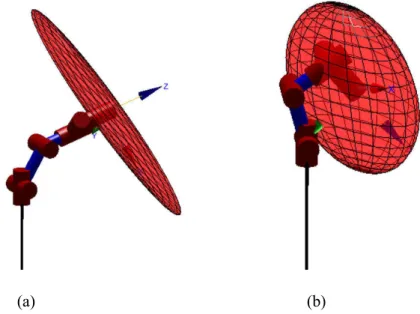Figure 3.3 Manipulability ellipsoid (a) W(Q) = 0.006 and (b) W(Q) = 0.0248 (Corke 2011) The damping factor is adjusted with respect to the manipulability index

### Summary of the Method

A bound on the feedback gain that allows the algorithm to remain stable was proposed by (Falco and Natale 2011).

### Example

34;Introduction to Inverse Kinematics with Jacobian Transpose, Pseudoinverse, and Damped Least Squares Methods." IEEE Transactions on Robotics. 34;Inverse Kinematic Solutions with Discrete Stability for Robot Manipulator Control." Journal of Dynamic Systems, Measurement and Control.

34; Resolved rate-of-motion control features of mechanisms, including self-propulsion." Acta Polytechnica Journal of Advanced Engineering. 34; Applications of damped least-squares methods for velocity-resolved and acceleration-resolved control of manipulators. " Journal of Dynamic Systems, Measurement and Control.

34;Resolved Motion Rate Control of Manipulators and Human Prostheses." IEEE Transactions on Human-Machine Systems.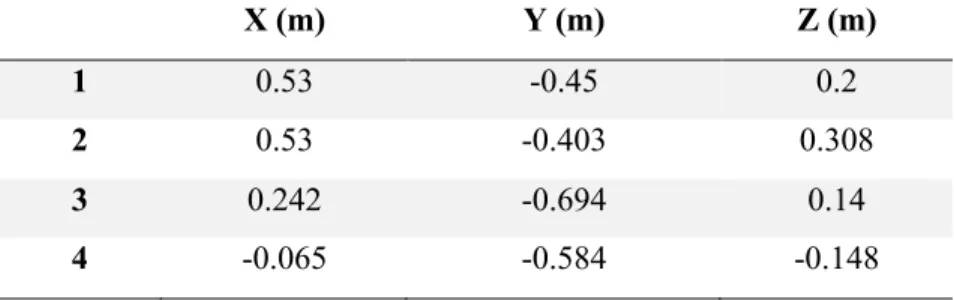Figure 3.8 Robot workcell showing both the virtual and real frame

## CASE STUDY

### Experimental Setup

The Neuromeka Indy 7 device was used for the experiments, whose modified Denavit Hartenberg parameters are shown in Figure 4.2. To determine the transformation matrix that relates the robot base to the work object frame, two sets of data are obtained, one in the robot base frame using the robot learning pendant and the other in the work object frame. To test the algorithm, the robot's task is shown in Figure 4.3 (b), and its waypoints in the frame of the virtual work object are listed in Table 4.2.

The transformation matrix describing the virtual work object frame relative to the actual work object frame. The waypoints in the actual work object frame are listed in Table 4.3. Table 4.3 Robot task path points in the actual work object frame. Plot the desired path and the feasible path in Figure 4.4 and insert the error between the feasible path and the desired path.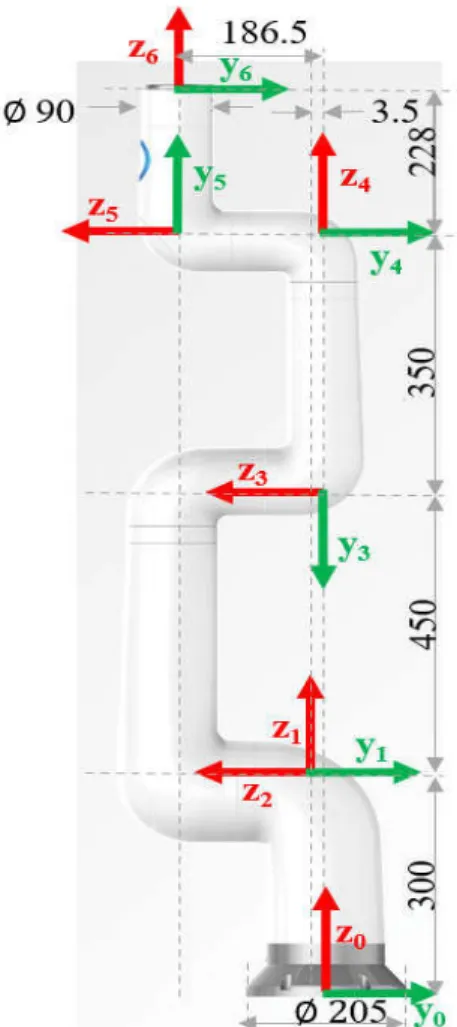Figure 4.2 Indy 7 modified DH Parameters (dimension in mm and angles in degree) Modified DH parameters

### Experiments

Despite the popularity of offline programming, accuracy remains a problem in this field due to the dimensional differences that occur in the real robot work cell, hence the need for robot program calibration. In this thesis, a method of adaptive calibration of the robotic program is developed that calculates the dimensional changes that occur in the real frame of the work object. The method developed in this thesis consists of two main steps, first we calculate the transformation matrix between the robot base and the real work object frame in order to regenerate a robot program in the real work object frame.

In future works, a method to calibrate the robot program including obstacle avoidance in the path regeneration algorithm will be studied. 34; Review of Pseudoinverse Control for Use with Kinematically Redundant Manipulators." IEEE Transactions on Systems, Man, and Cybernetics. 34;Coordinated Resolved Motion Control of Closed-Chain Bilateral Manipulators." International Journal of Advanced Robotic Systems 13(3): 80.

34;An Online Relative Position and Orientation Error Calibration Methodology for Work Cell Robot Operations.” Robotics Computer-Integrated Manufacturing.

## CONCLUSION AND FUTURE RESEARCH

### Conclusion

Offline programming allows users to reprogram the robot without stopping the production process. Calibration is required for the robot program to determine the actual positions of the robot and its peripherals within the workcell. This process must be completed before the program is loaded into a real system, as dimensional differences between real and virtual workcells can cause critical TCP position errors.

A review of current work cell calibration methods includes methods that identify dimensional variations in the actual work object either using a robot as a measuring tool or other measuring sensors such as laser and vision. To compensate for dimensional variations, the actual values ​​are then loaded into offline programming software to recreate a new robot program. By using the manipulability index as a measure of how close the robotic manipulator is to the singular region, the singularity can be avoided, but at the cost of the end effector deviating from the desired path.

The experimental results showed that the end-effector position error introduced by the damping factor is not bounded, which may lead the robot manipulator to miss its target position while avoiding a singularity.

Future Research

수치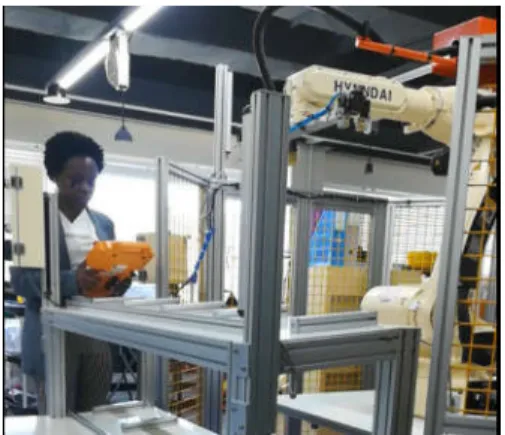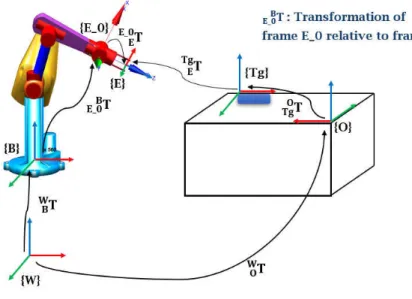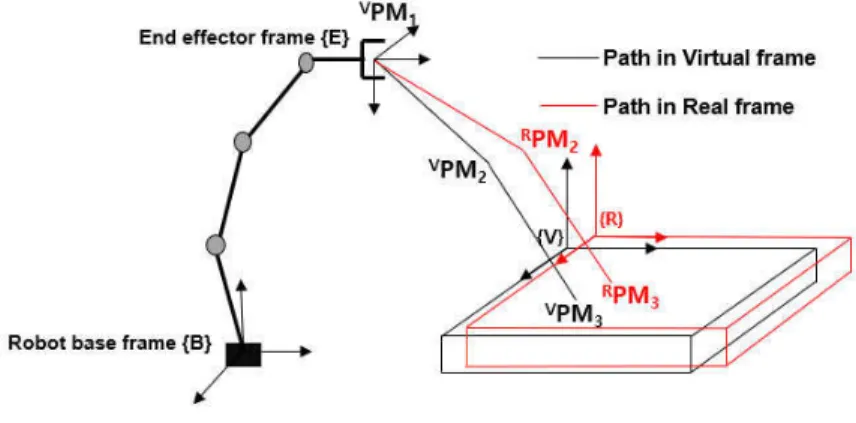+7

참조

관련 문서

First Deputy Prime Minister and Minister of Defense, Sheikh Nasser Al-Sabah discussed with UK Minister of International Development Roy Stuart the possibility

Effect of pH on the Stability of PPE-loaded Liposomes Although the anionic and neutral liposomes did not show significant differences in mean sizes upon storage at

generalization of the exponential integral and to further derive some inequalities for the generalized function2. The results are presented in

One possible way of solving these equations is to write the blade equations in the ﬁxed reference frame using Fourier coordinate transformation, and then solve

In 2015, New South Wales Aboriginal Affairs, Department of Education (‘NSW Aboriginal Affairs’) engaged the Australian Institute of Health and Welfare (AIHW) to provide an overview

The Australian Institute of Health and Welfare (AIHW) annually collects data from organisations funded by the Australian Government to provide one or more of the following

There are three broad options for the United States to address the North Korean threat without the use of force: (1) return to negotiations without preconditions, which

Atmospheric surface activity concentrations of cesium and xenon measured at 11 stations (Figure 1) operated by the Comprehensive Nuclear-Test- Ban Treaty Organization (CTBTO),

For the local registration, three uncorrected fiducials close to a target were used to determine the transformation from the working frame to the destination frame.. For example, in

언어 선택하기

웹사이트는 선택한 언어로 번역됩니다.

기타 언어